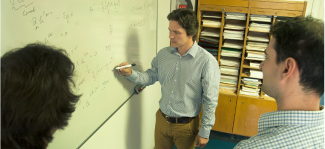### ## Theoretical PhysicsDepartment leader György Wolf The mission of the Department of the Theoretical Physics is the theoretical investigation of the fundamental questions of nature. There are three major research areas at the department: quantum field theory, particle and heavy ion physics and gravitation. Part of the research is directly related to ongoing experiments of heavy ions, particle physics (LHC, RHIC) and gravitational wave detection (VIRGO). The list of current research topics includes the following: phenomenological and theoretical study on relativistic heavy ion collisions; non-extensive thermodynamics; integrable classical and quantum field theories; quantum symmetries; extended objects in field theories; analytical and numerical studies of Einstein’s equations, problems related to gravitational wave detection.

#### Particle Physics and Field Theory Research Group

Our investigations are directed at the general mathematical (symplectic, canonical, Hamiltonian, …) structure, radiating modes, and conserved quantities of field theoretical and dynamical systems. Our aim is the exploration of their exceptional and particular properties applying mathematical methods...

#### Heavy-ion Physics

High-energy heavy-ion physics is connected to a large variety of physics disciplines, therefore researches made into this direction possess a large variety. The research directions range from the fundamental concepts of classical and modern thermodynamics, hydrodynamics, and quantum theory to perturbative and non-perturbative QCD, high-energy nuclear effects, hadronization and hadron phenomenology...

#### Holographic Quantum Field Theory Research Group

According to the holographic conjuncture, our universe of three space dimensions and one time dimension might be equivalent to the four+one dimension universe, containing only gravity. We would be the holograms of four-dimensional objects in the gravitational universe - on its three-dimensional edge -and everything we do could be explained through higher- dimension gravitational processes...

#### Gravitational Physics Research Group

The members of the Gravitational Physics Research Group are involved in various research activities including field theory, numerical and post-Newtonian general relativity, experimental gravitational wave data analysis and fundamental research in algorithm optimization and many-core computer science.